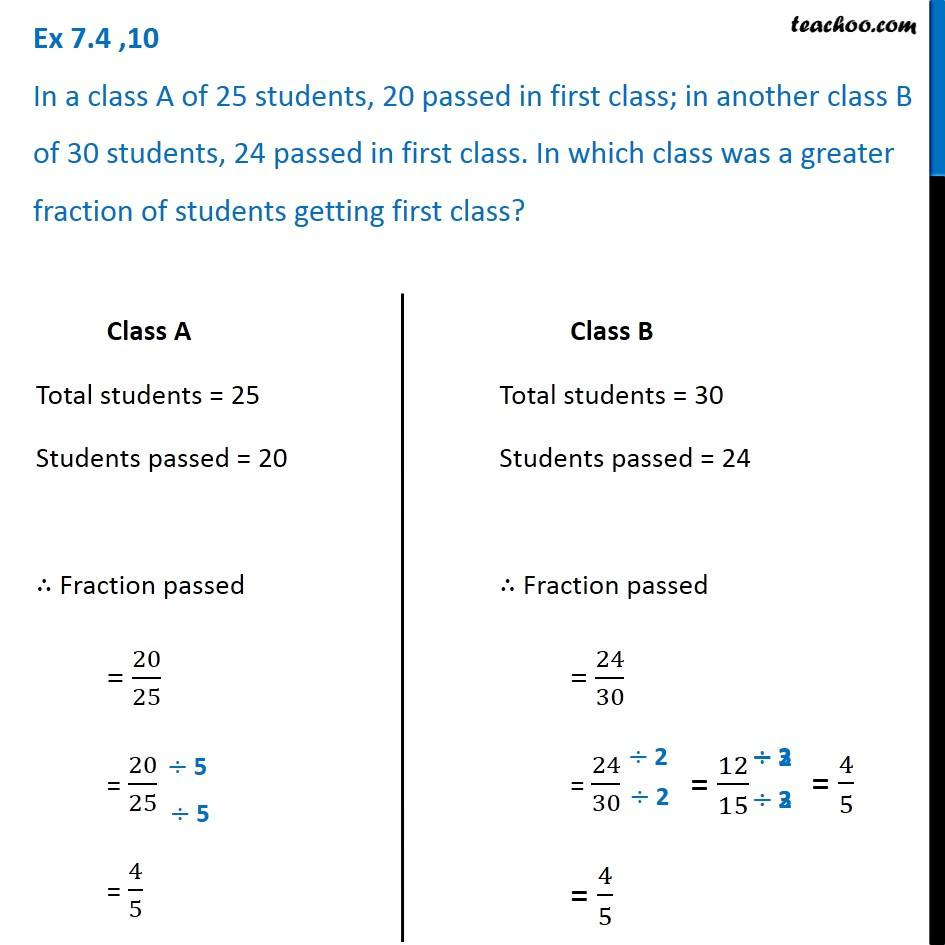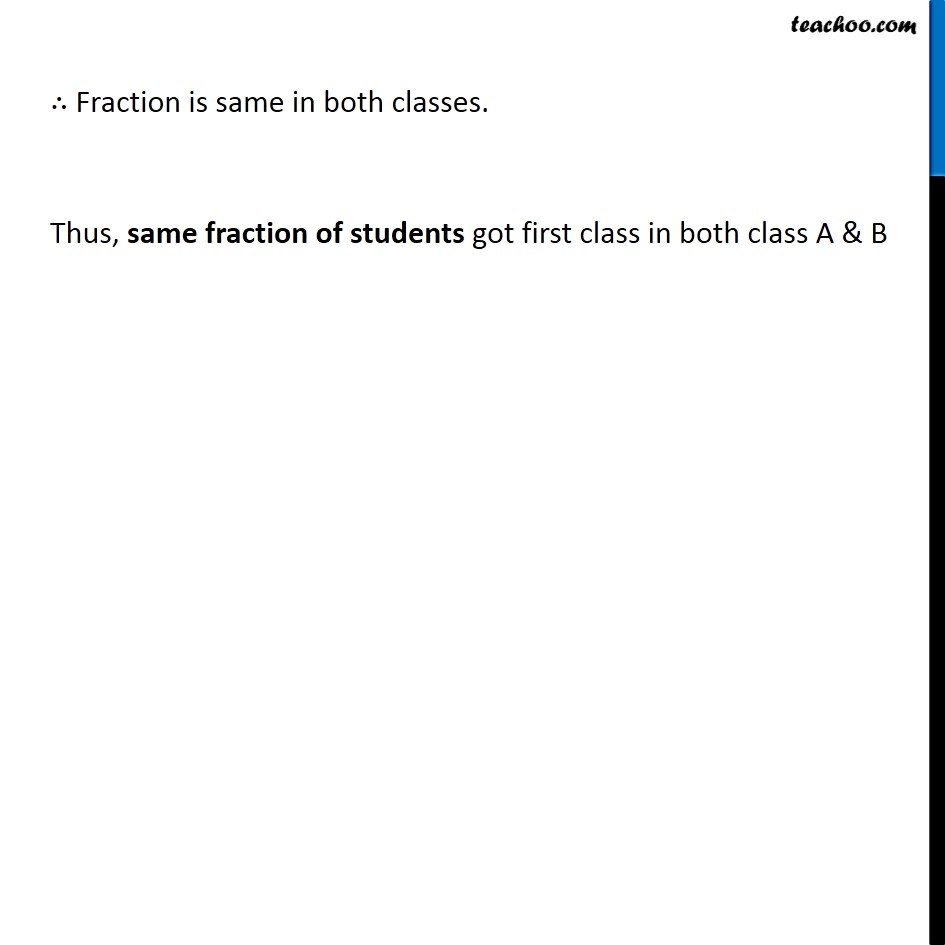Ex 7.4

Chapter 7 Class 6 Fractions
Serial order wiseLearn in your speed, with individual attention - Teachoo Maths 1-on-1 Class

### Transcript

Ex 7.4 ,10 In a class A of 25 students, 20 passed in first class; in another class B of 30 students, 24 passed in first class. In which class was a greater fraction of students getting first class? Class A Total students = 25 Students passed = 20 ∴ Fraction passed = 20/25 = 20/25 = 4/5 Class B Total students = 30 Students passed = 24 ∴ Fraction passed = 24/30 = 24/30 = 4/5 ∴ Fraction is same in both classes. Thus, same fraction of students got first class in both class A & B